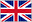# MAT SAT

0    19 flashcards

QuestionAnswerIntegers
Positive and negative whole numbers, and zero; NOT fractions or decimals
Prime Numbers
An integer that has exactly two distinct factor: itself and 1. All prime numbers are positive; the smallest prime number is 2. One is not prime
Rational Numbers
All positive and negative integers, fractions, and decimal numbers; technically, any number that can be expressed as a fraction of two integers - which means everything except numbers containing weird radicals such as (pi) or e or square root of 2.
Irrational Numbers
Any number that does not end or repeat (in other words, any number that isn't rational). This includes all numbers with radicals that can't be simplified, such as square root. Also all numbers containing PI or E. Note that repeating decimals like 0.3333... are rational
Real Numbers
Any number on the number line; everything except imaginary numbers.
Imaginary Numbers
The square roots of negative numbers, that is, any numbers containing i, which represents square root of -1
Consecutive Numbers
The members of a set listed in order, without skipping any; consecutive integers: -3, -2, -1, 0, 1, 2; consecutive positive multiples of 3: 3,6,9,12
Distinct Numbers
Numbers that are different from each other.
Sum
Difference
The result of subtracting numbers
Product
The result of multiplying numbers
Quotient
The result of dividing numbers
Remainder
The integer left over after dividing two numbers. On the MSAT a remainder is ALWAYS an integer
Reciprocal
The result when 1 is divided by a number. For example, the reciprocal of 3/4 is 4/3.
Positive Difference
The number you get by subtracting the smaller of two numbers from the bigger one.
Absolute Value
The positive version of a number.
Arithmetic Mean
The average of a list of values; also simply referred to as the 'mean'
Median
The middle value in a list when arranged in increasing order; in a list with an even number of members, the average of two middle values
Mode
The value that occurs most often in a list.